# RS Aggarwal Solutions for Class 6 Maths Chapter 14 Constructions (Using Ruler and a Pair of Compasses)

RS Aggarwal Solutions for Class 6 Maths Chapter 14 Constructions (Using Ruler and a Pair of Compasses) are provided here in PDF format. Students can go through RS Aggarwal Solutions for better understanding about the topics discussed in the chapter. To score good marks in the exams, students are advised to practice RS Aggarwal textbook questions to clear all their doubts and boost their self confidence in Mathematics.

RS Aggarwal textbook questions are solved by the experts at BYJU’S in simple PDF format with good explanation. Download PDF of Class 6 Chapter 14 in their respective links.

## Download PDF of RS Aggarwal Solutions for Class 6 Maths Chapter 14 Constructions (Using Ruler and a Pair of Compasses)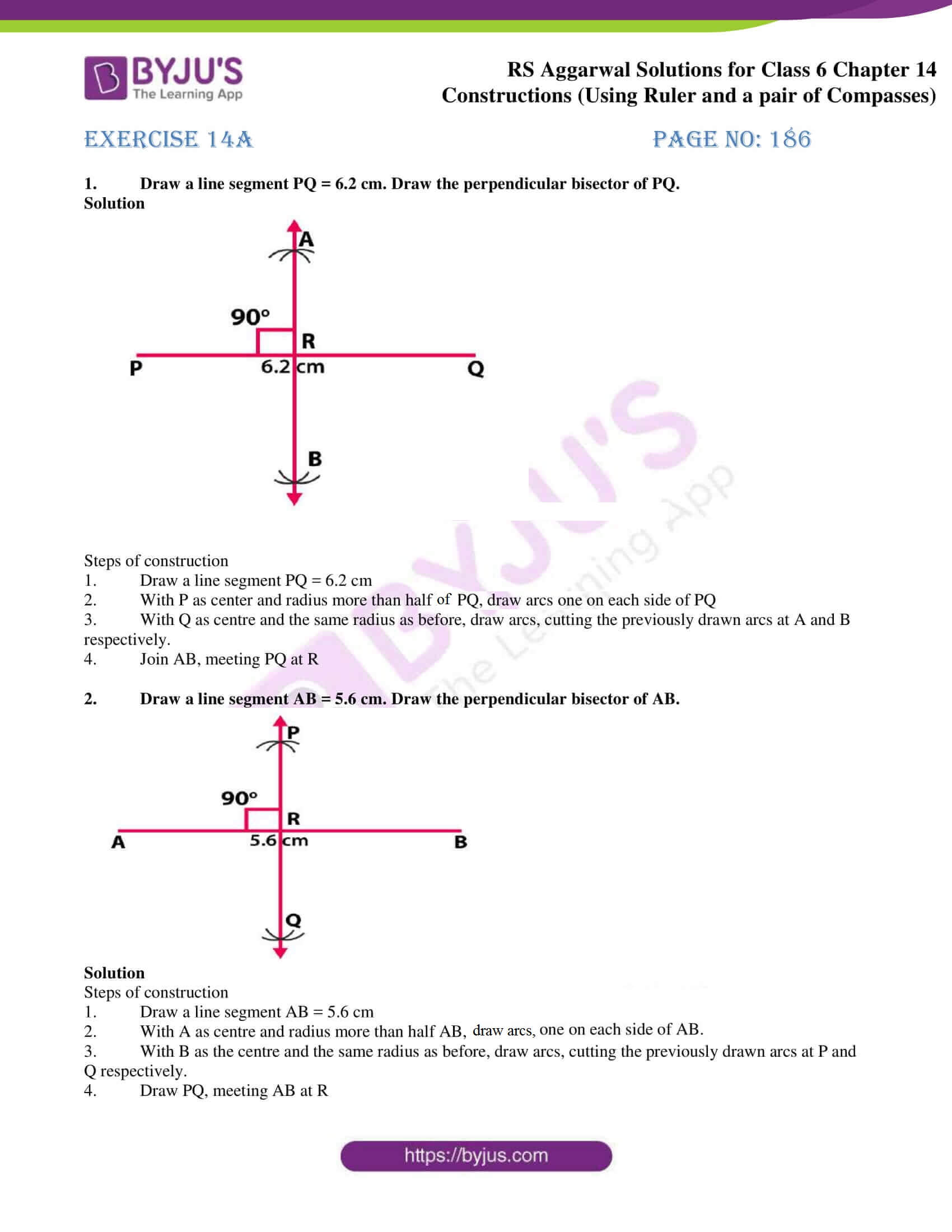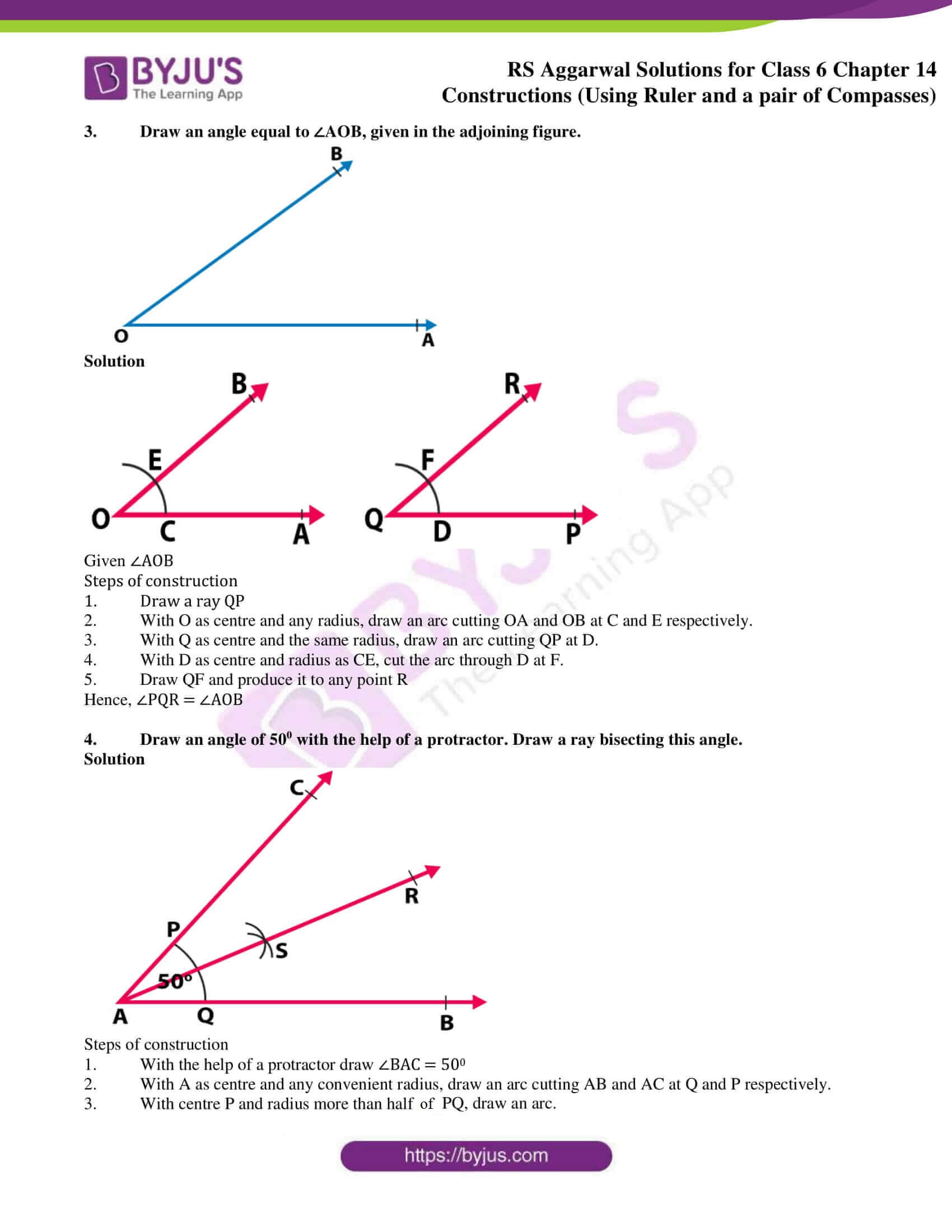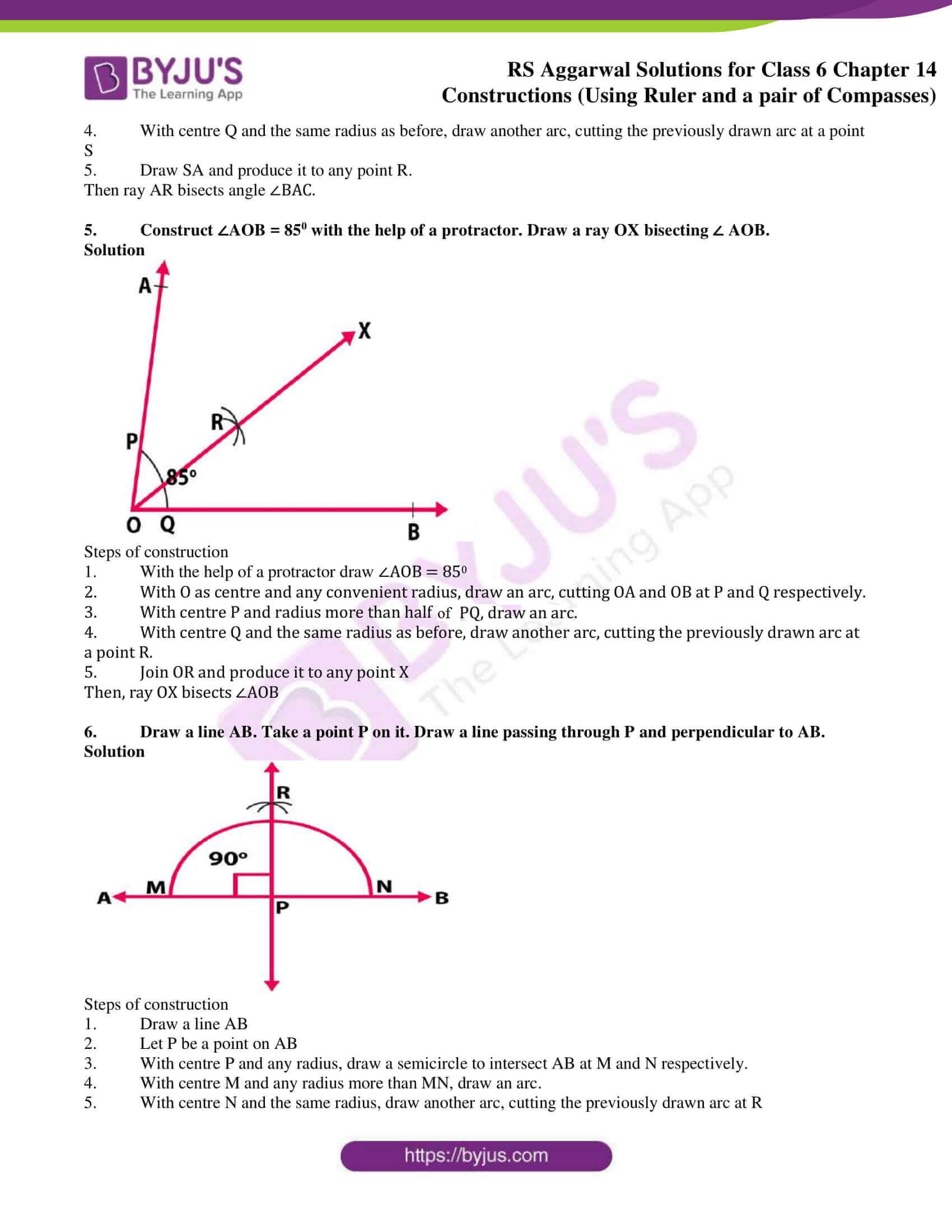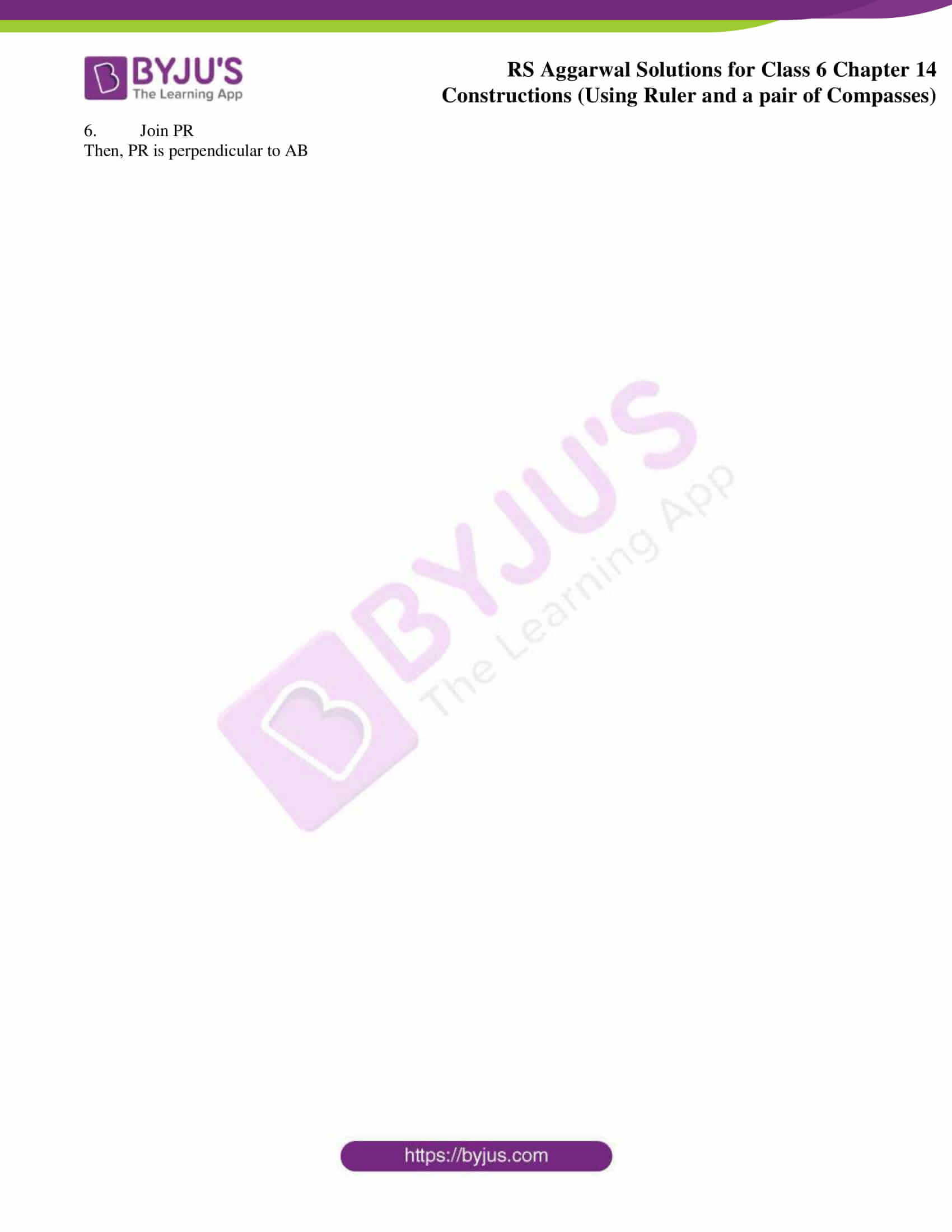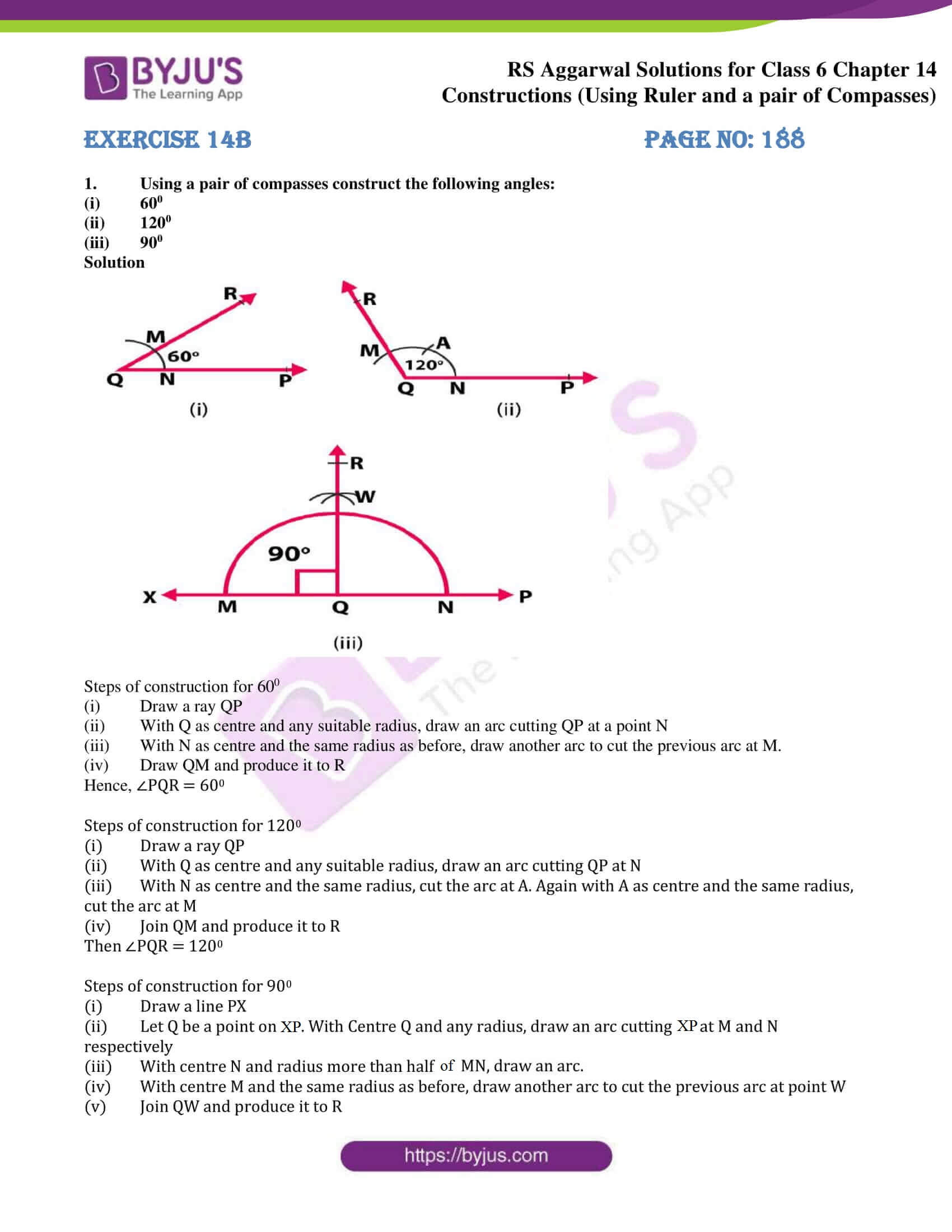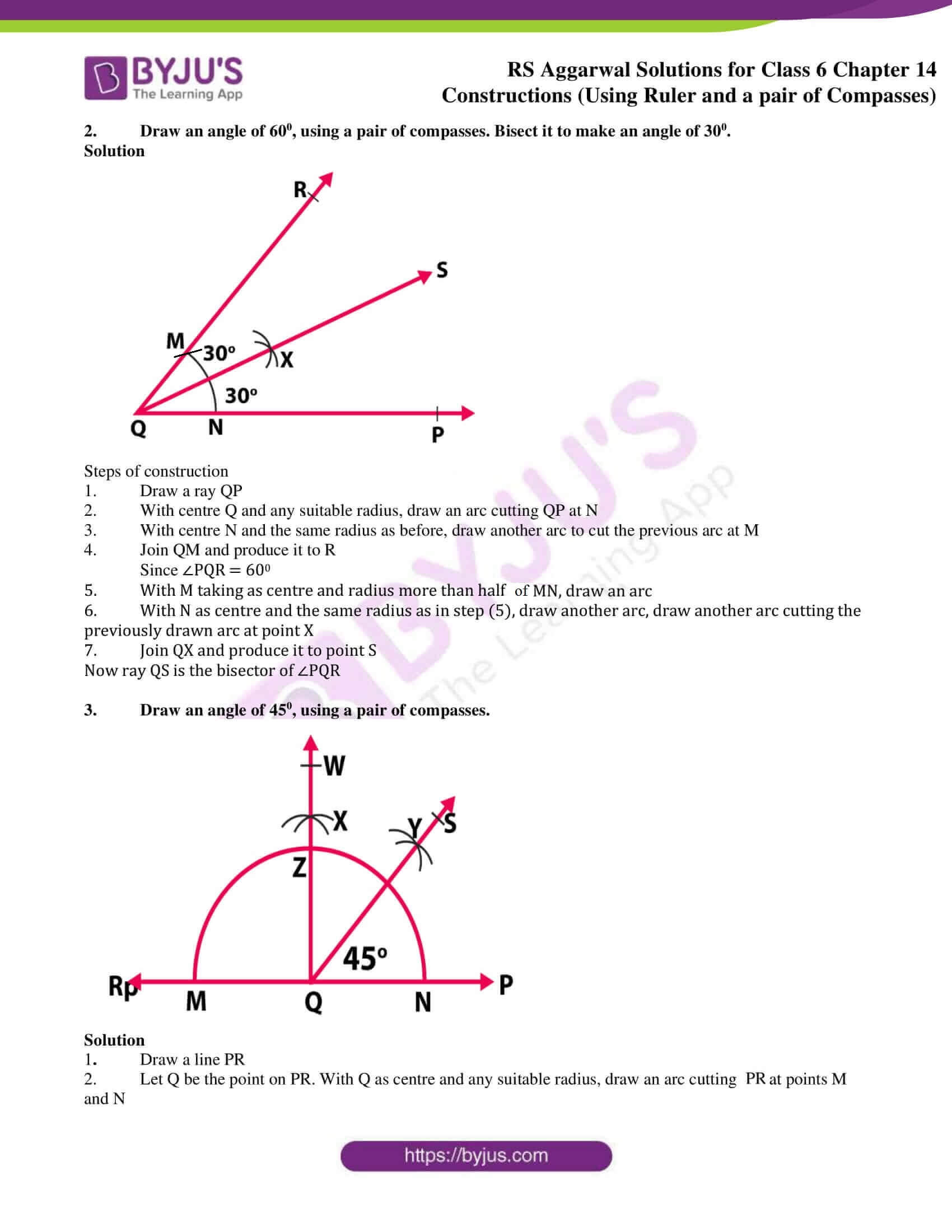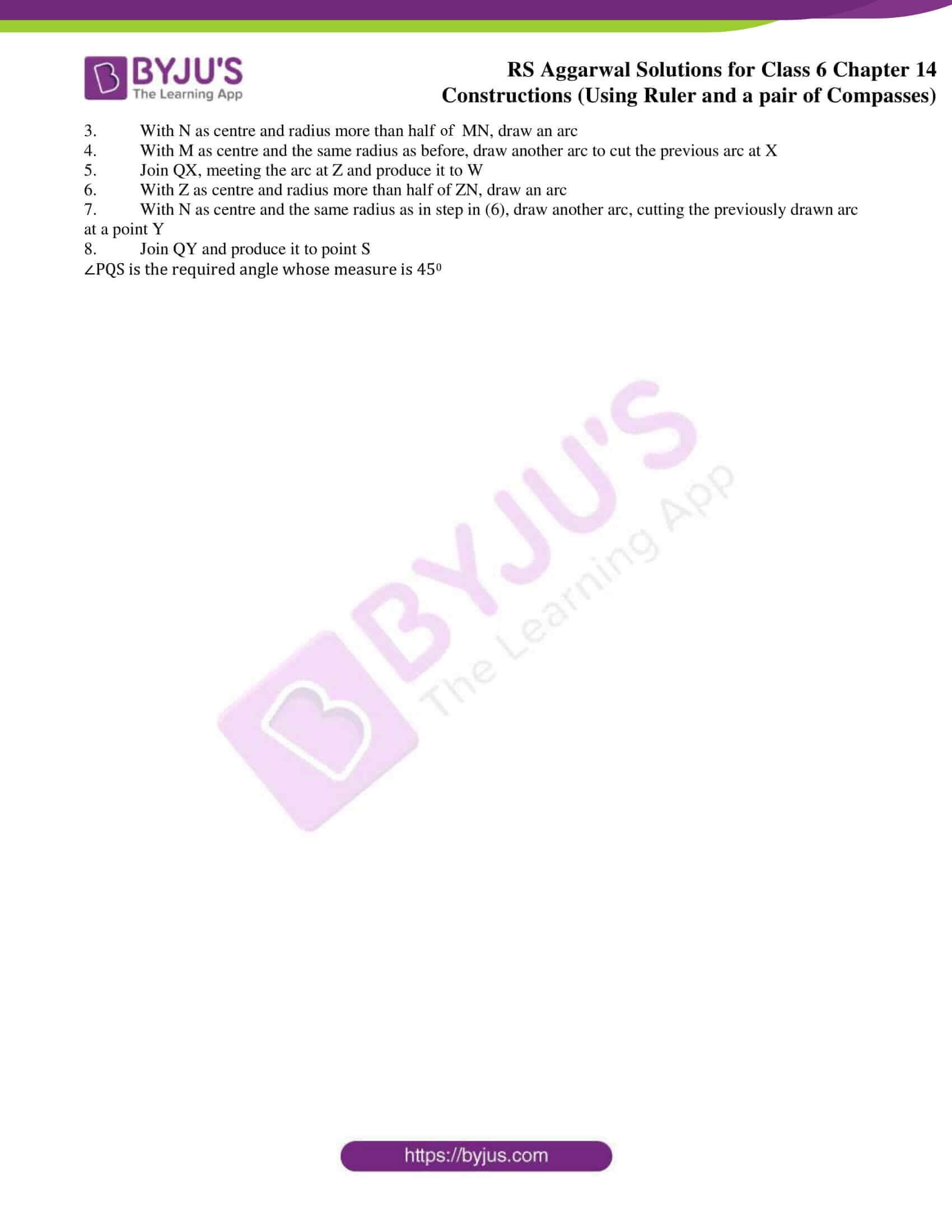### Access answers of RS Aggarwal Solutions for Class 6 Maths Chapter 14 – Constructions (Using Ruler and a Pair of Compasses)

Exercise 14A PAGE nO: 186

1. Draw a line segment PQ = 6.2 cm. Draw the perpendicular bisector of PQ.

Solution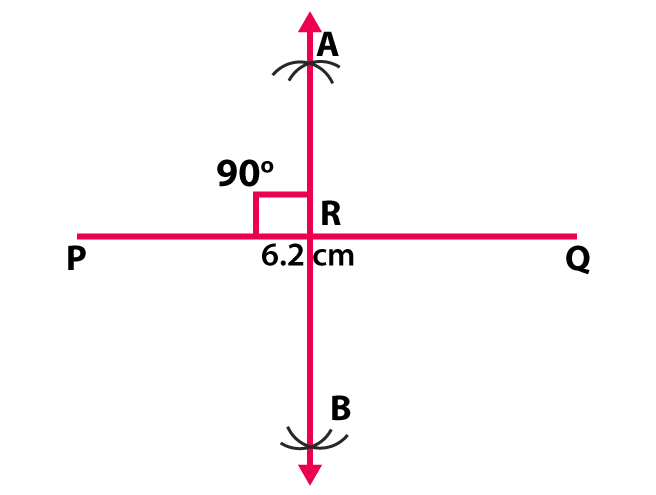Steps of construction

1. Draw a line segment PQ = 6.2 cm

2. With P as center and radius more than half of PQ, draw arcs one on each side of PQ

3. With Q as centre and the same radius as before, draw arcs, cutting the previously drawn arcs at A and B respectively.

4. Join AB, meeting PQ at R

2. Draw a line segment AB = 5.6 cm. Draw the perpendicular bisector of AB.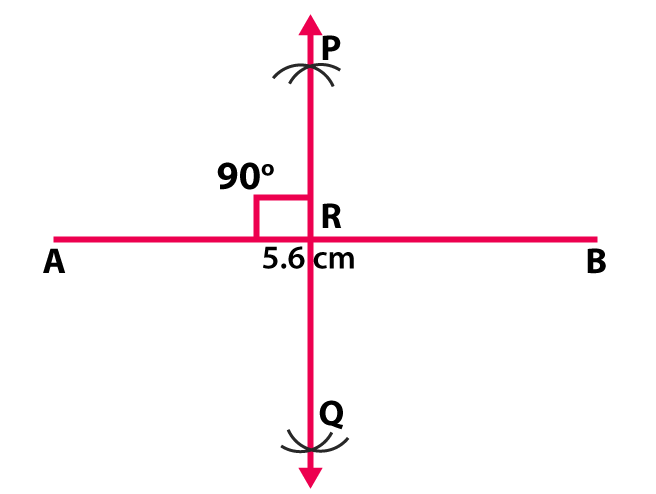Solution

Steps of construction

1. Draw a line segment AB = 5.6 cm

2. With A as centre and radius more than half of AB, draw arcs, one on each side of AB.

3. With B as the centre and the same radius as before, draw arcs, cutting the previously drawn arcs at P and Q respectively.

4. Draw PQ, meeting AB at R

3. Draw an angle equal to ∠AOB, given in the adjoining figure.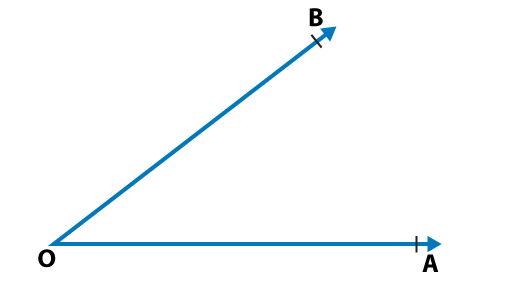Solution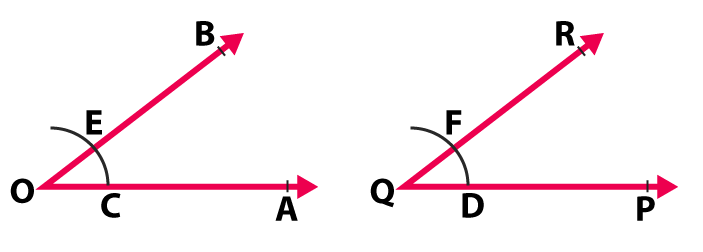Given ∠AOB

Steps of construction

1. Draw a ray QP

2. With O as centre and any radius, draw an arc cutting OA and OB at C and E respectively.

3. With Q as centre and the same radius, draw an arc cutting QP at D.

4. With D as centre and radius as CE, cut the arc through D at F.

5. Draw QF and produce it to any point R

Hence, ∠PQR = ∠AOB

4. Draw an angle of 500 with the help of a protractor. Draw a ray bisecting this angle.

Solution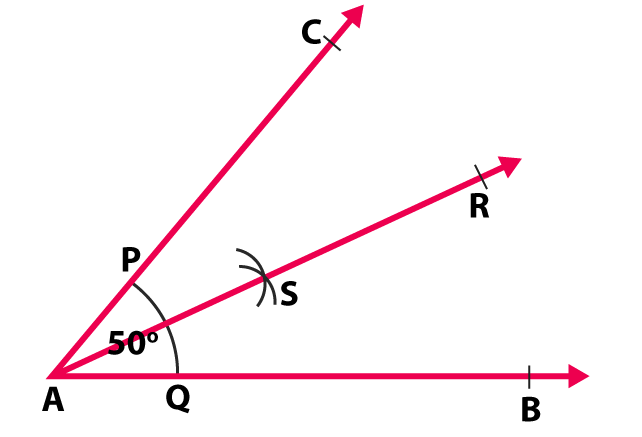Steps of construction

1. With the help of a protractor draw ∠BAC = 500

2. With A as centre and any convenient radius, draw an arc cutting AB and AC at Q and P respectively.

3. With centre P and radius more than half of PQ, draw an arc.

4. With centre Q and the same radius as before, draw another arc, cutting the previously drawn arc at a point S

5. Draw SA and produce it to any point R.

Then ray AR bisects angle ∠BAC.

5. Construct ∠AOB = 850 with the help of a protractor. Draw a ray OX bisecting ∠ AOB.

Solution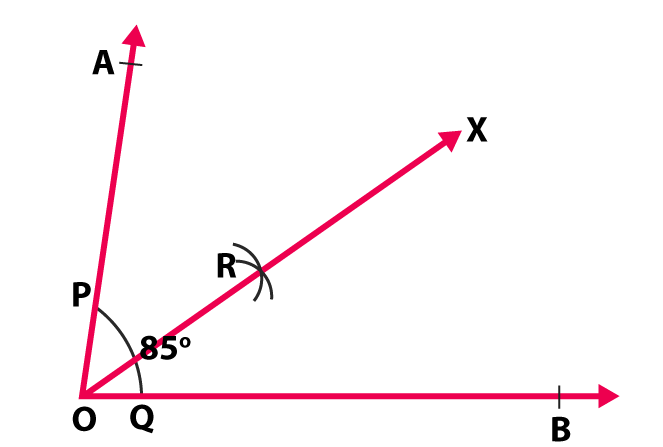Steps of construction

1. With the help of a protractor draw ∠AOB = 850

2. With O as centre and any convenient radius, draw an arc, cutting OA and OB at P and Q respectively.

3. With centre P and radius more than half of PQ, draw an arc.

4. With centre Q and the same radius as before, draw another arc, cutting the previously drawn arc at a point R.

5. Join OR and produce it to any point X

Then, ray OX bisects ∠AOB

6. Draw a line AB. Take a point P on it. Draw a line passing through P and perpendicular to AB.

Solution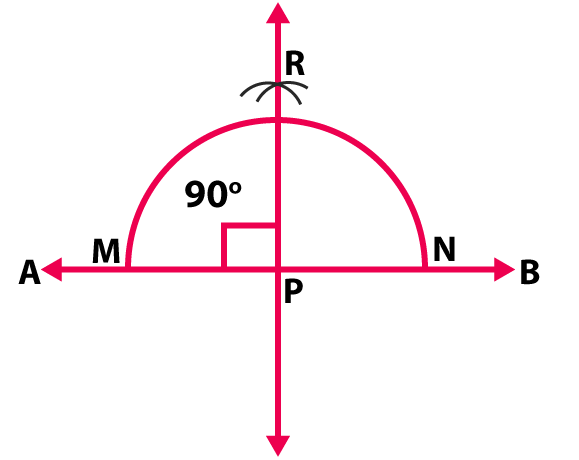Steps of construction

1. Draw a line AB

2. Let P be a point on AB

3. With centre P and any radius, draw a semicircle to intersect AB at M and N respectively.

4. With centre M and any radius more than MN, draw an arc.

5. With centre N and the same radius, draw another arc, cutting the previously drawn arc at R

6. Join PR

Then, PR is perpendicular to AB

Exercise 14B PAGE No: 188

1. Using a pair of compasses construct the following angles:

(i) 600

(ii) 1200

(iii) 900

Solution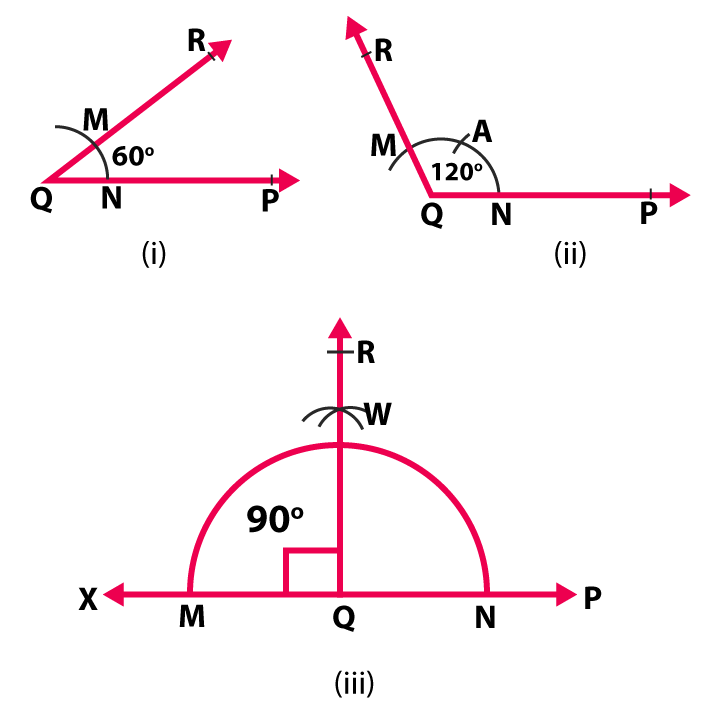Steps of construction for 600

(i) Draw a ray QP

(ii) With Q as centre and any suitable radius, draw an arc cutting QP at a point N

(iii) With N as centre and the same radius as before, draw another arc to cut the previous arc at M.

(iv) Draw QM and produce it to R

Hence, ∠PQR = 600

Steps of construction for 1200

(i) Draw a ray QP

(ii) With Q as centre and any suitable radius, draw an arc cutting QP at N

(iii) With N as centre and the same radius, cut the arc at A. Again with A as centre and the same radius, cut the arc at M

(iv) Join QM and produce it to R

Then ∠PQR = 1200

Steps of construction for 900

(i) Draw a line PX

(ii) Let Q be a point on XP. With Centre Q and any radius, draw an arc cutting XP at M and N respectively

(iii) With centre N and radius more than half of MN, draw an arc.

(iv) With centre M and the same radius as before, draw another arc to cut the previous arc at point W

(v) Join QW and produce it to R

2. Draw an angle of 600, using a pair of compasses. Bisect it to make an angle of 300.

Solution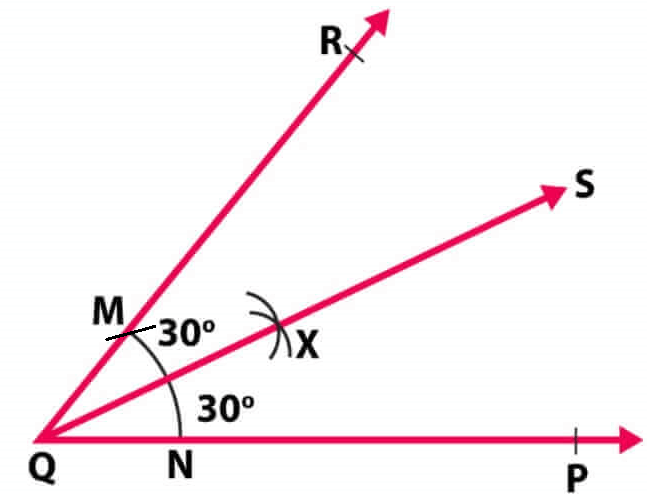Steps of construction

1. Draw a ray QP

2. With centre Q and any suitable radius, draw an arc cutting QP at N

3. With centre N and the same radius as before, draw another arc to cut the previous arc at M

4. Join QM and produce it to R

Since ∠PQR = 600

5. With M taking as centre and radius more than half of MN, draw an arc

6. With N as centre and the same radius as in step (5), draw another arc, draw another arc cutting the previously drawn arc at point X

7. Join QX and produce it to point S

Now ray QS is the bisector of ∠PQR

3. Draw an angle of 450, using a pair of compasses.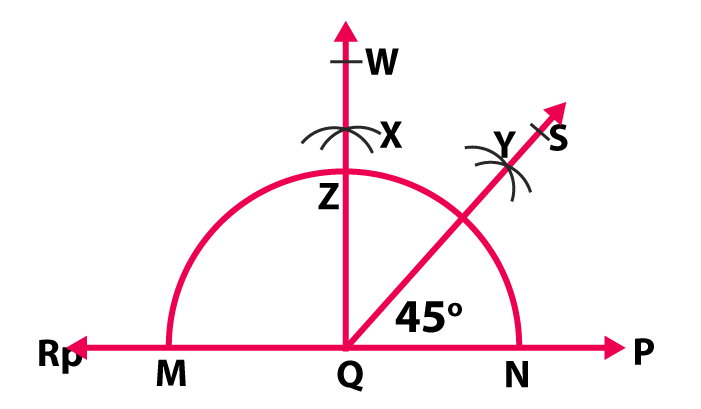Solution

1. Draw a line PR

2. Let Q be the point on PR. With Q as centre and any suitable radius, draw an arc cutting PR at points M and N

3. With N as centre and radius more than half of MN, draw an arc

4. With M as centre and the same radius as before, draw another arc to cut the previous arc at X

5. Join QX, meeting the arc at Z and produce it to W

6. With Z as centre and radius more than half of ZN, draw an arc

7. With N as centre and the same radius as in step in (6), draw another arc, cutting the previously drawn arc at a point Y

8. Join QY and produce it to point S

∠PQS is the required angle whose measure is 450

### RS Aggarwal Solutions for Class 6 Maths Chapter 14 Constructions (Using Ruler and a Pair of Compasses)

Chapter 14 – Constructions (Using Ruler and a Pair of Compasses) includes 2 exercises. Each and every question has been solved comprehensively. Let’s have a glance at the topics which are included in this chapter of RS Aggarwal Class 6.

• To draw the perpendicular bisector of a given line segment
• To draw an angle equal to the given angle
• To bisect a given angle
• To draw a line perpendicular to a given line from a point on it
• To draw a line perpendicular to a given line from a point outside it
• To draw a line parallel to a given line through a point outside it
• Construction of some special angles using a pair of compasses.

Also, access RS Aggarwal Solutions for Class 6 Chapter 14 Exercises

Exercise 14A

Exercise 14B

### Chapter Brief of RS Aggarwal Solutions for Class 6 Maths Chapter 14 – Constructions (Using Ruler and a Pair of Compasses)

Here students are taught about Construction using ruler and compasses. Students are advised to draw constructing a perpendicular bisector, constructing an angle bisector, to draw a line perpendicular to a given line from a point on it and construction of some special angles using a pair of compasses.